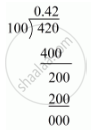# Express the Following Rational Number as Decimal: 42/100 - Mathematics

Express the following rational number as decimal:

42/100

#### Solution

Given rational number is 42/100

Now we have to express this rational number into decimal form. So we will use long division method as below.Hence, 42/100=0.42

Is there an error in this question or solution?
Chapter 1: Number Systems - Exercise 1.2 [Page 13]

#### APPEARS IN

RD Sharma Mathematics for Class 9
Chapter 1 Number Systems
Exercise 1.2 | Q 1.1 | Page 13
Share